﻿ 一个钙振荡模型的分支分析 Bifurcation Analysis of a Calcium Oscillation Model

Vol.04 No.01(2015), Article ID:14833,7 pages
10.12677/AAM.2015.41002

Bifurcation Analysis of a Calcium Oscillation Model

Min Shentu, Yu Chang*

Department of Mathematics, College of Science , Beijing University of Chemical Technology, Beijing

Received: Jan. 22nd, 2015; accepted: Feb. 6th, 2015; published: Feb. 13th, 2015ABSTRACT

The bifurcation of a calcium oscillation model is investigated by applying the center manifold theorem and the bifurcation theory. We theoretically analyze the existence of Hopf bifurcation. The numerical analysis results suggest that the quasi-periodic oscillation in this model results from torus bifurcation. Numerical simulation also shows some new complex dynamics, such as torus bifurcation and cyclic fold bifurcation which are different from the results before.

Keywords:Bifurcation, The Center Manifold, Calcium Oscillation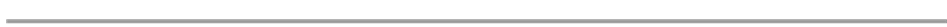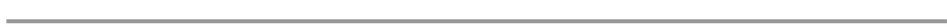1. 引言

2. 钙振荡模型(1)3. 平衡点的稳定性和局部分支

(1) 当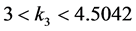时，系统(1)有一个稳定的结点；

(2) 当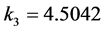时，系统(1)有一个非双曲平衡点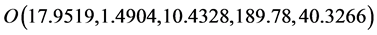，其特征值为：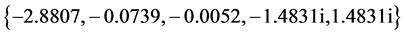(3) 当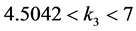时，系统(1)有一个鞍点。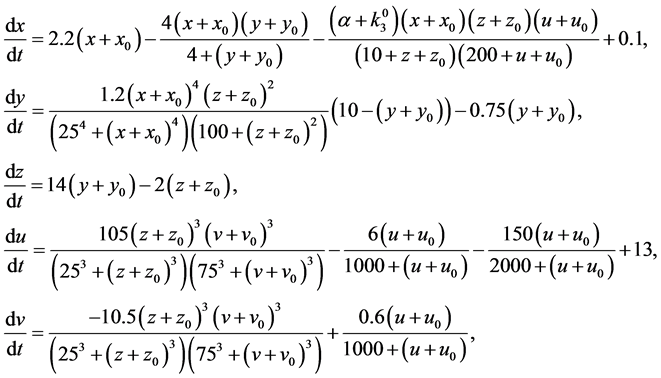(2)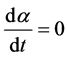(3)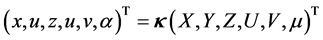，则系统(2)~(3)可变换成系统(4)：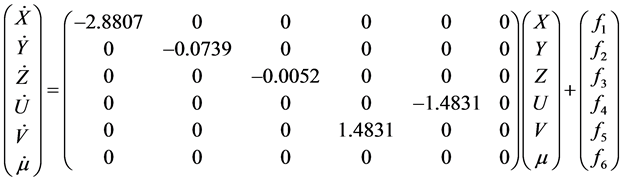(4)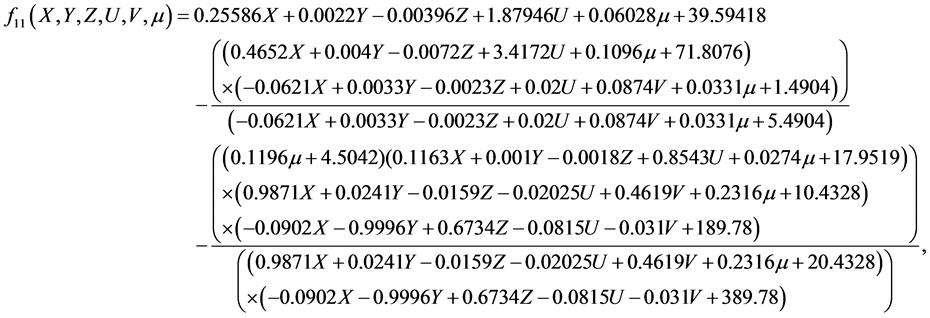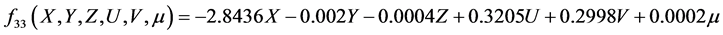(5)(6)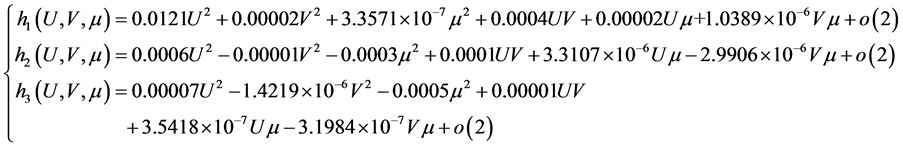(7)(8)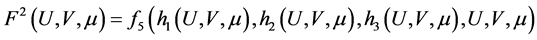4. 数值模拟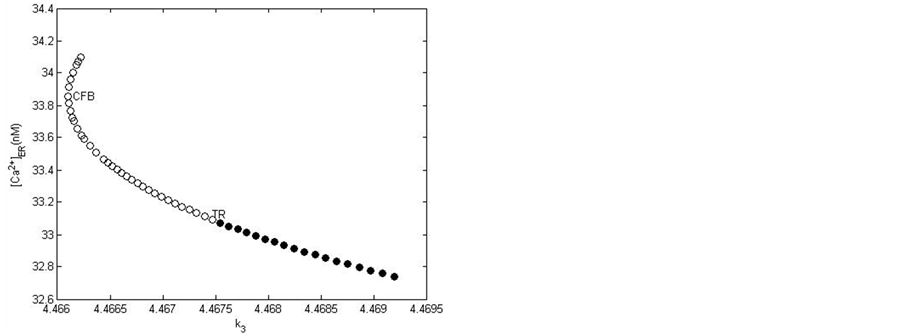Figure 1. The bifurcation diagram and local amplificationTable 1. Bifurcation points and k3 values in Figure 1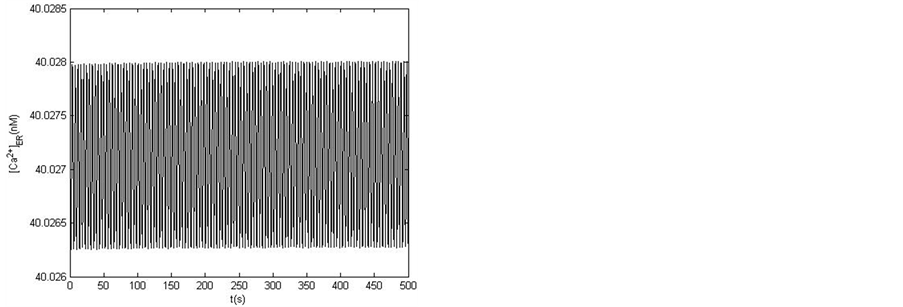Figure 2. Quasi-periodic orbit and its time series for k3 = 4.467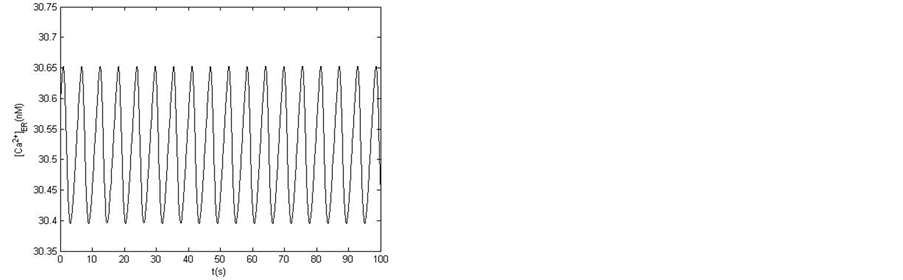Figure 3. Stable periodic orbit and its time series for k3 = 4.505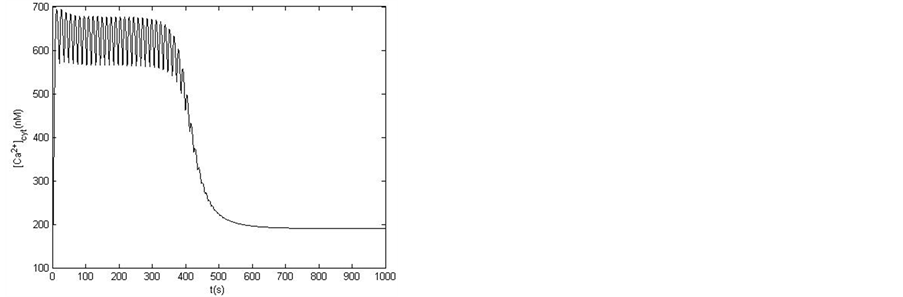Figure 4. Simulated orbit in the article 

5. 结论

Bifurcation Analysis of a Calcium Oscillation Model. 应用数学进展,01,7-14. doi: 10.12677/AAM.2015.41002

1. 1. Cuthbertson, K.S.R. and Chay, T.R. (1991) Modelling receptor-controlled intracellular calcium oscillators. Cell Calcium, 12, 97-109.

2. 2. Wang, J.H., Huang, X.D. and Huang, W.D. (2007) A quantitative kinetic model for ATP-induced intracellular Ca2+ oscillations. Journal of Theoretical Biology, 245, 510-519.

3. 3. Wiggins, S. (1990) Introduction to applied nonlinear dynamical systems and chaos. Springer, Berlin.